Science, Maths & Technology

### Become an OU studentGeometry

Start this free course now. Just create an account and sign in. Enrol and complete the course for a free statement of participation or digital badge if available.

# 1 Angles

## 1.1 Angles, notation and measurement

In everyday language, the word ‘angle’ is often used to mean the space between two lines (‘The two roads met at a sharp angle’) or a rotation (‘Turn the wheel through a large angle’). Both of these senses are used in mathematics, but it is probably easier to start by thinking of an angle in terms of the second of these – as a rotation.

The diagram below shows a fixed arm and a rotating arm (with the arrow), which are joined together at O, forming an angle between them. Imagine that the rotating arm, which is pivoted at O, initially rests on top of the fixed arm and that it then rotates in the direction of the arrow. Focus on the size of the marked angle between the arms.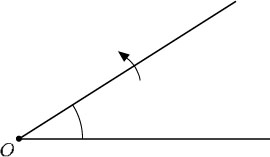At first the angle is quite sharp, but it becomes less so. It then becomes a right angle, and subsequently gets much blunter until the two arms form a straight line. Then it starts to turn back upon itself, passing through a three-quarter turn and, when the rotating arm gets back to the start, it rests on top of the fixed arm again.

The most common unit for expressing angles is degrees, denoted by °, with a complete turn or revolution being equal to 360°. Angles can also be measured in radians, and you will meet this unit of measure if you study further maths, science or technology courses.

### Acute angle

Any angle that is less than a quarter turn; that is, less than 90°. An example of an acute angle is the angle that a door makes with a doorframe when it is ajar.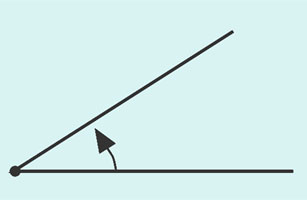Figure ang1

### Right angle

The angle that corresponds to a quarter turn; it is exactly 90°. The angles at the corners of most doors, books and windows are right angles.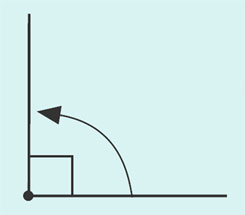Figure ang2

### Obtuse angle

Any angle that is between a quarter turn and a half turn; that is, between 90° and 180°. An example is the angle between the blades of a pair of scissors when they are open as wide as possible.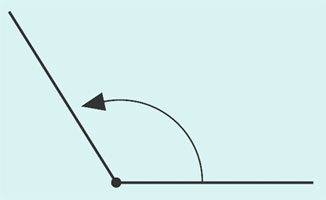Figure ang3

### Half turn (Straight angle)

This corresponds to a straight line; it is exactly 180°. The pages of an open book that is lying flat approximately describe a half turn.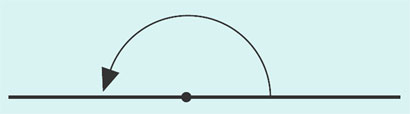### Reflex angle

Any angle that is between a half turn and a complete turn; that is, between 180° and 360°. When a box is opened and the hinged lid falls back so as to rest on the surface on which the box is standing, the angle that the lid turns through is a reflex angle.

### Complete turn

This corresponds to a complete turn, or one revolution; it is exactly 360°. This is the angle that the minute hand of a clock turns through in an hour.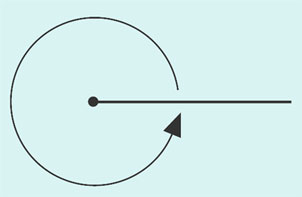Figure ang6

Remember that if the angle between two straight lines is 90°, then the lines are said to be perpendicular to each other.

Sometimes it is necessary to refer to a turn that is more than one complete revolution, and so is greater than 360°. An example is the angle that the minute hand of a clock turns through in a period of 12 hours: each complete revolution of the minute hand amounts to 360°, so twelve revolutions amount to 12 × 360° = 4320°.

Several different notations are used for labelling angles. For example, the angle below can be referred to as ‘angle BAC’ and written as BC or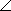BAC, or it can be referred to as the angle ‘theta’ and labelled θ.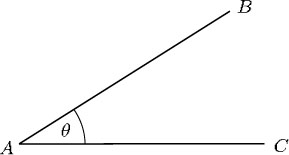Alternatively, an angle may be denoted by the label on the vertex but with a hat on it. The vertex is another name for the ‘corner’ of an angle. For instance, the angle θ above may be denoted by, which is read as ‘angle A’.

This notation can be ambiguous if there is more than one angle at the vertex, as in the example below.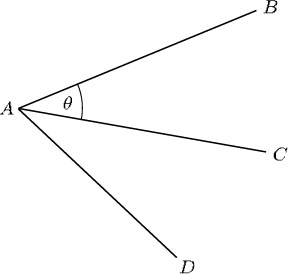In such cases, θ can be specified as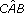,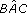,CAB orBAC – the middle letter indicates the vertex and the two outer letters identify the ‘arms’ of the angle.